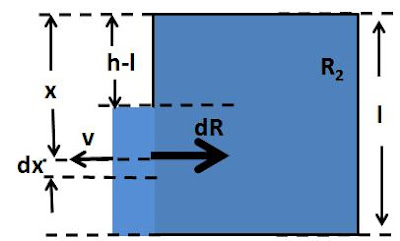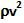## Friday, May 29, 2009

### Irodov Problem 1.327Consider an infinitesimally thin section of water of width dx escaping out of and at depth x. Let the velocity of this flow be v. The reaction pressure exerted by this section of flowing water is given by. The cross section of this infinitesimally thin section of water flow is bdx. Thus, the reaction force exerted by the infinitesimally thin section of flow is given by. Similar to as solved in Problem 1.327,Hence we have,